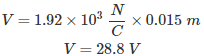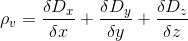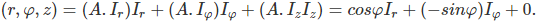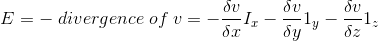# MCQs on Electromagnetic Fields

##### Page 10 of 20. Go to page 1 2 3 4 5 6 7 8 9 10 11 12 13 14 15 16 17 18 19 20
01․ An electric field of 1.92 × 103 N/C is maintained across two plates separated by 0.015 m. Potential difference across plates will be
1.4 V.
2.88 V.
28.8 V.
14.40 V.

From, V = E.d02․ Which of the following statement is incorrect?
A conductor is an equipotential body and E is always tangential to the conductor.
In a liner dielectric P varies linearly with E.
A dielectric material is liner if ε does not change with applied field.
A dielectric material is isotropic if ε does not change with direction.

E is normal to conductor.

03․ Between a hollow and solid metal sphere, charge reside
on outer surface in hollow and throughout in solid
on outer surface in both.
on outer surface in hollow and throughout in solid.
None of above.

Charge always reside outer surface of any object.

04․ The electric flux is given D = (2y2 + z) Ix + 4xy Iy + x Iz C/m2. The volume charge density at point (-1, 0, 3) is
0 C/m3.
- 2 C/m3.
- 4 C/m3.
- 8 C/m3.

Divergence of D = ρ v05․ Gradient of scalar field is expressed as
Outward flux of a vector field per unit volume as the volume about the point tends to zero.
Gradient of divergence of a vector field minus the curl of the vector field.
Circulation of a vector field per unit area as the area tends to zero.
Maximum rate of increase of scalar function at a point.

Gradient is operation performed on a saclar function which result in a vector function and it is the maximum rate of increase of the scalar function at a point.

06․ A vector field A = p In is given in Cartesian coordinates. In cylindrical coordinates it will be represented as
A = cosφ Ir.
A = cosφ Ir + sinφ Iφ.
A = sinφ Ir.
A = cosφ Ir - sinφ Ir.07․ A potential field is given by V = 3x2 y – yz. The electric field at P(2, -1, 4) is
12 Ix - 8 Iy V/m.
12 Ix - Iz V/m.
12 Ix + 8 Iy + Iz V/m.
12 Ix - 8 Iy - Iz V/m.

E = - divergence v08․ A sphere of radius with a uniform charge density ρv C/m3 shall have electric flux density at radius “r = a” equal to
v/4 C/m2.
v/3 C/m2.
v C/m2.
ρv/3 C/m2.

By Gauss’s law, Surface integral of (D.ds) = volume integral of ρv dv) Or, D.4π a2 = 4 π a3 ρv/3.

09․ An infinite sheet has a charge density of 150 μ C/m. The flux density in μ C/m 2 is
50.
75.
100.
1/75.

For infinite sheet, | E | = σ/(2 × εo) | D | = σ/2 = 150/2 = 75 μC/m2.

10․ An infinite non conducting sheet of charge has σ of 10-7 c/m3. Equipotential surface for a potential of 10 volts is.
0.
0.88 mm.
1.32 mm.
1.77 mm.

E = σ/(2 × εo) V = E.x. C = V/E = V × εo/ σ.

<<<89101112>>>